• 累计撰写 145 篇文章
• 累计创建 86 个标签
• 累计收到 22 条评论

### 目 录CONTENT# 关于Queue你需要知道的2022-03-26 / 0 评论 / 0 点赞 / 250 阅读 / 2,321 字 / 正在检测是否收录...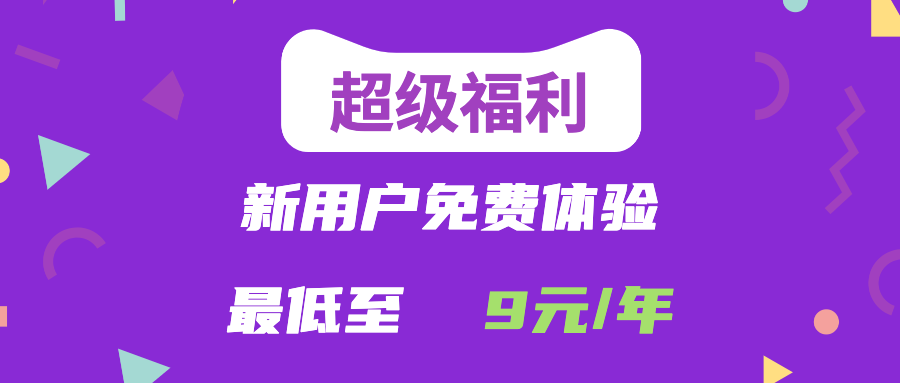广告

# 前言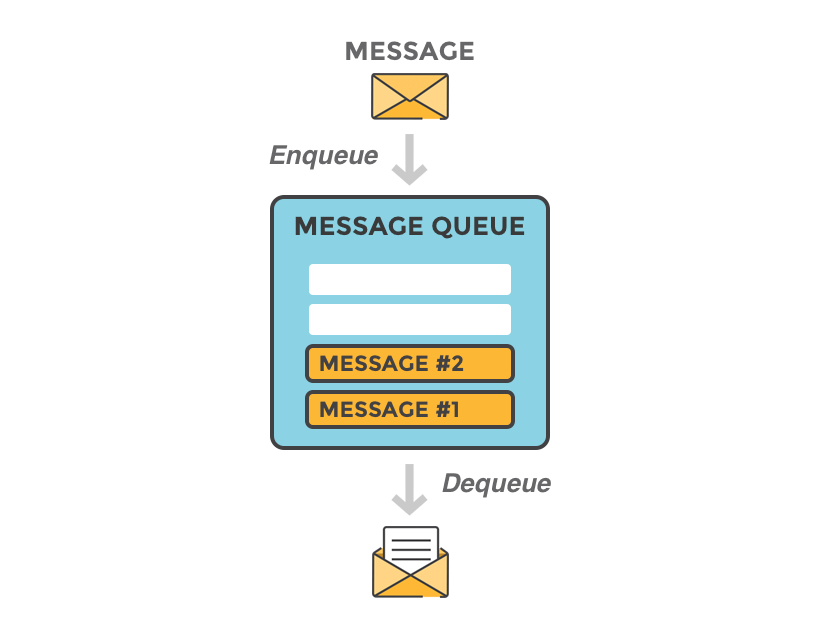# 关于 Queue

QueuePython 内置的，线程安全的队列库。其默认支持队列同步，实现了先入先出、后入先出和优先级队列。Queue 在多线程通信中使用甚广。

# 队列实践

## 先进先出（FIFO）

from queue import Queue

def BanZhuan(q):
print('我开始搬砖了！')
for i in range(5):
print(f'搬砖信息：砖-{i} --> 小推车')

# 将砖放到队列中
q.put(f'砖-{i}')

def LeiZhuan(q):
print('我开始垒砖了！')
while True:

# 从队列中取砖
zhuan = q.get()

# 如果队列空了（砖搬完了），就跳出循环
if q.empty():
break
print(f'垒砖信息：{zhuan} --> 墙')

if __name__ == '__main__':

# 创建队列
q=Queue(10)

# 起一个线程进行搬砖

# 起一个线程进行垒砖

# 开始搬砖&垒砖
t1.start()
t2.start()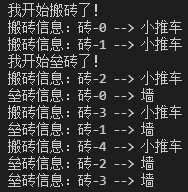## 后进先出（LIFO）

from queue import Queue,LifoQueue
import time,random

def BanZhuan(q):
print('我开始搬砖了！')
for i in range(5):
print(f'搬砖信息：砖-{i} --> 小推车')

# 将砖放到队列中
q.put(f'砖-{i}')

def LeiZhuan(q):
print('我开始垒砖了！')
while True:

# 从队列中取砖
zhuan = q.get()
time.sleep(random.random())
# 如果队列空了（砖搬完了），就跳出循环
if q.empty():
break
print(f'垒砖信息：{zhuan} --> 墙')

if __name__ == '__main__':

# 创建队列
q=LifoQueue(10)

# 起一个线程进行搬砖

# 起一个线程进行垒砖

# 开始搬砖&垒砖
t1.start()
t2.start()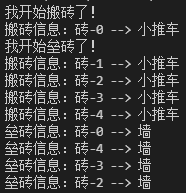## 优先级队列（PriorityQueue）

from queue import Queue,LifoQueue,PriorityQueue
import time,random

def BanZhuan(q):
print('我开始搬砖了！')
for i in range(5):
print(f'搬砖信息：砖-{i} --> 小推车')

# 将砖放到队列中,优先级队列传入一个元组，第一个元素为优先级，越小优先级越高
q.put((100-i,f'砖-{i}'))

def LeiZhuan(q):
print('我开始垒砖了！')
while True:

# 从队列中取砖
zhuan = q.get()
time.sleep(random.random())
print(f'垒砖信息：{zhuan} --> 墙')

# 如果队列空了（砖搬完了），就跳出循环
if q.empty():
break

if __name__ == '__main__':

# 创建队列
q=PriorityQueue(10)

# 起一个线程进行搬砖

# 起一个线程进行垒砖

# 开始搬砖&垒砖
t1.start()
t2.start()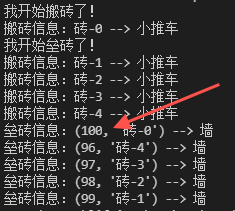...
def LeiZhuan(q):
print('我开始垒砖了！')
while True:
time.sleep(0.1)
# 从队列中取砖
zhuan = q.get()
time.sleep(0.1)
print(f'垒砖信息：{zhuan} --> 墙')

# 如果队列空了（砖搬完了），就跳出循环
if q.empty():
break
...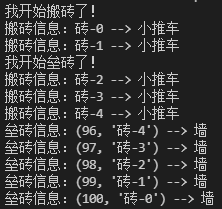0
1.2.广告
• 0
1.2.